# 12. You need 500 mls of a HEPES buffered saline solution containing 21 mM HEPES, 137 mM NaCI, 5 mM KCI, 50 mM sodiu...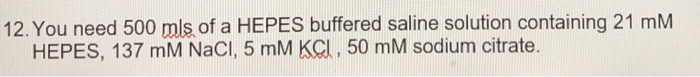12. You need 500 mls of a HEPES buffered saline solution containing 21 mM HEPES, 137 mM NaCI, 5 mM KCI, 50 mM sodium citrate.

The necessary moles of each compound are calculated to prepare the solution:

n HEPES = M * V = 0.021 M * 0.5 L = 0.0105 mol

n NaCl = 0.137 M * 0.5 L = 0.0685 mol

n KCl = 0.005 M * 0.5 L = 0.0025 mol

n Citrate = 0.05 M * 0.5 L = 0.025 mol

The required grams of each compound are calculated, according to its molar weight:

g HEPES = n * MM = 0.0105 mol * 238.3 g / mol = 2.50 g

g NaCl = 0.0685 mol * 58.44 g / mol = 4.00 g

g KCl = 0.0025 mol * 74.55 g / mol = 0.19 g

g Citrate = 0.025 mol * 258.1 g / mol = 6.45 g

If you liked the answer, please rate it in a positive way, you would help me a lot, thank you.

##### Add Answer of: 12. You need 500 mls of a HEPES buffered saline solution containing 21 mM HEPES, 137 mM NaCI, 5 mM KCI, 50 mM sodiu...
Similar Homework Help Questions
• ### A) Prepare 50 mL of a NaCI solution containing 1 ppm Na. - first prepare 250 mL of a 500 ppm Na solution in a 250 mL volumetric flask - dilute 1/500 to create 50 mL of 1 ppm Na solution in a 50...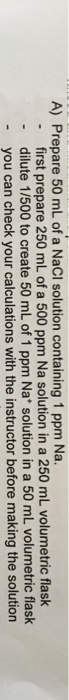A) Prepare 50 mL of a NaCI solution containing 1 ppm Na. - first prepare 250 mL of a 500 ppm Na solution in a 250 mL volumetric flask - dilute 1/500 to create 50 mL of 1 ppm Na solution in a 50 mL volumetric flask - you can check your calculations with the instructor before making the solution A) Prepare 50 mL of a NaCI solution containing 1 ppm Na. - first prepare 250 mL of a 500...

• ### 3. Severely dehydrated patients may be given a "physiological buffered saline" (PBS) solution wit...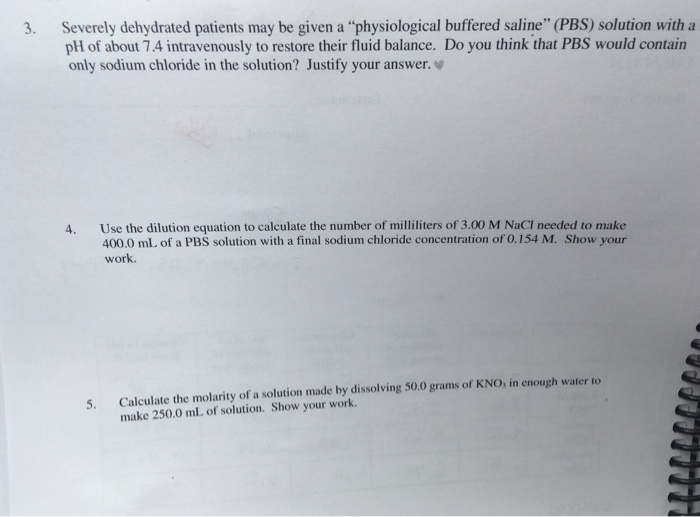3. Severely dehydrated patients may be given a "physiological buffered saline" (PBS) solution with a pH of about 7.4 intravenously to restore their fluid balance. Do you think that PBS would contain only sodium chloride in the solution? Justify your answer. 4. Use the dilution equation to calculate the number of milliliters of 3.00 M NaCI needed to make 400.0 mL of a PBS solution with a final sodium chloride concentration of 0.154 M. Show your work. 5. Calculate the...

• ### Question 2 1 pts You need to make a 500 mL buffer containing 35 mM NaCl...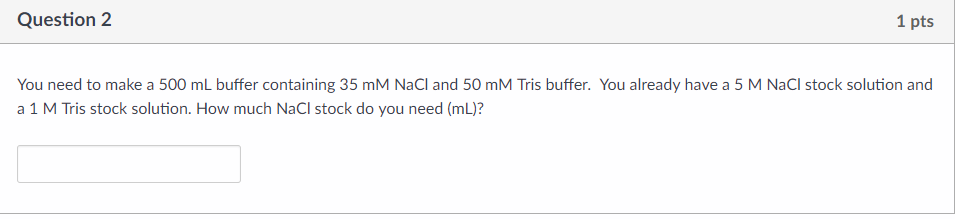Question 2 1 pts You need to make a 500 mL buffer containing 35 mM NaCl and 50 mM Tris buffer. You already have a 5 M NaCl stock solution and a 1 M Tris stock solution. How much NaCl stock do you need (mL)?

• ### Solutions practice problems. calculate how much of each ingredient you would need to make the solution...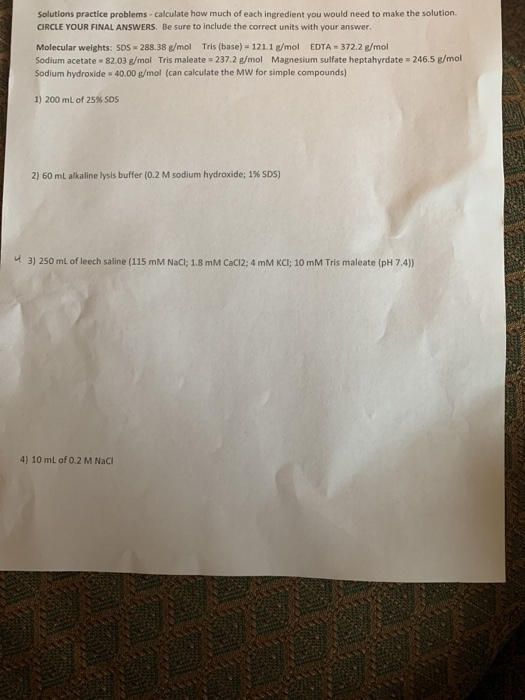Solutions practice problems. calculate how much of each ingredient you would need to make the solution CIRCLE YOUR FINAL ANSWERS. Be sure to include the correct units with your answer. Molecular weights: SDS-288.38 g/mol Tris (base) = 121.1 g/mol EDTA = 372.2 g/mol Sodium acetate 82.03 g/mol Tris maleate = 237.2 g/mol Magnesium sulfate heptahyrdate=246.5 g/mol Sodium hydroxide - 40.00 g/mol (can calculate the MW for simple compounds) 1) 200 mL of 25% SOS 2) 60 ml alkaline lysis buffer...

• ### Making Solutions Practice Problems: I. How would you prepare 100 ml of a 30% (w/v) solution...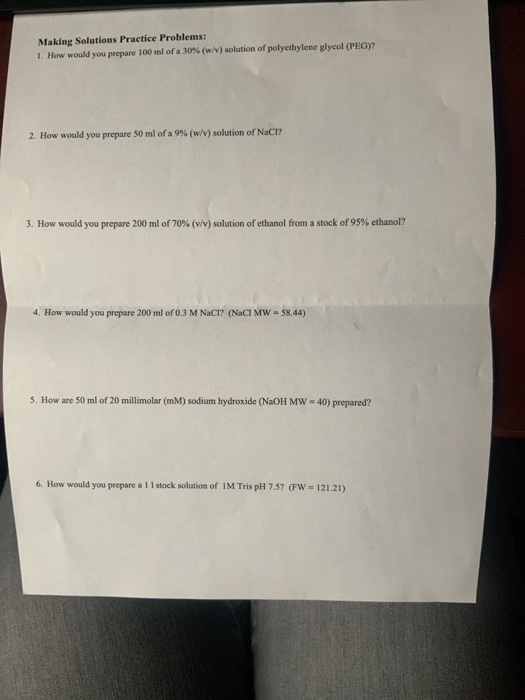Making Solutions Practice Problems: I. How would you prepare 100 ml of a 30% (w/v) solution of polyethylene glycol (PEG)? 2. How would you prepare 50 ml of a 9 % (w /v ) solution of NaCI? 3. How would you prepare 200 ml of 70 % ( v /v ) solution of ethanol from a stock of 95% ethanol? How would you prepare 200 ml of 0.3 M NaCl? (NaCl MW 58.44) 4. 5. How are 50 ml of...

• ### #5 please! thanks 3. A patient is receiving a normal saline solution, infusing continuously at 80...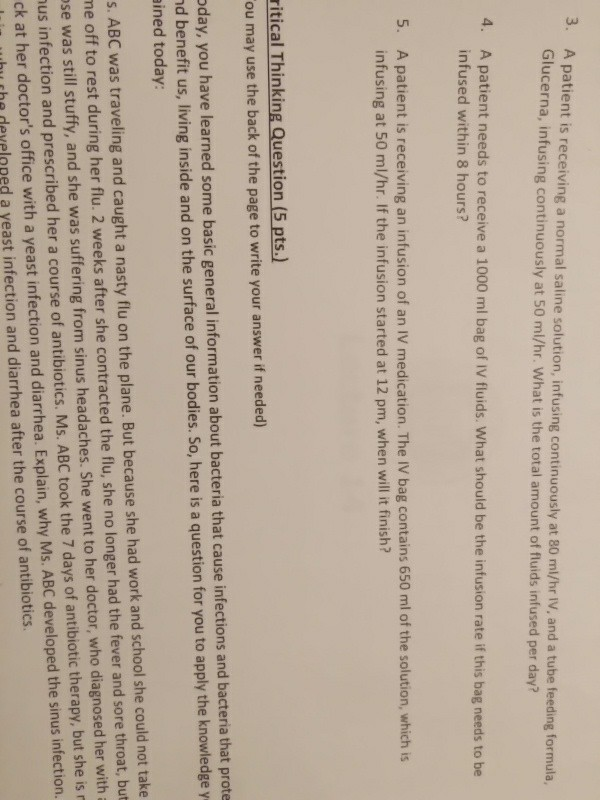#5 please! thanks 3. A patient is receiving a normal saline solution, infusing continuously at 80 ml/hr V, and a tube feeding formula ucerna, infusing continuously at 50 ml/hr. What is the total amount of fluids infused per day? Gl 4. A patient needs to receive a 1000 ml bag of IV fluids. What should be the infusion rate if this bag needs to be infused within 8 hours? 5. A patient is receiving an infusion of an IV medication....

• ### How many tablets will you give? 3 12. Order: 500 ml to infuse at 50 ml/hr....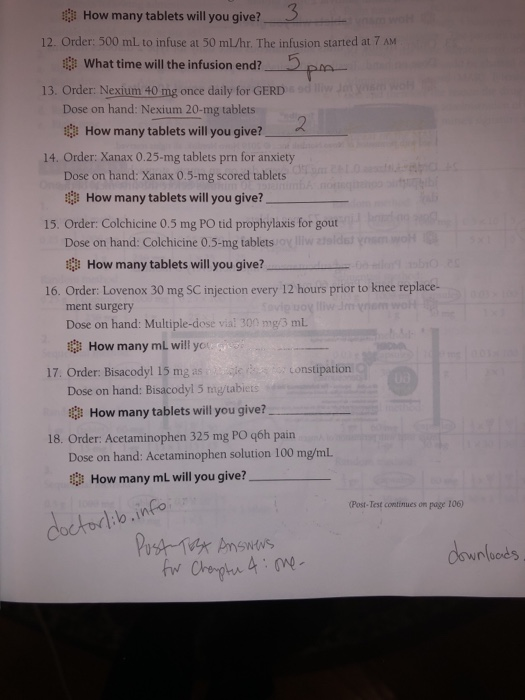How many tablets will you give? 3 12. Order: 500 ml to infuse at 50 ml/hr. The infusion started at 7 AM What time will the infusion end?_5pm 13. Order: Nexium 40 mg once daily for GERD Dose on hand: Nexium 20-mg tablets How many tablets will you give? 2. 14. Order: Xanax 0.25-mg tablets prn for anxiety ose on hand: Xanax 0.5-mg scored tablets How many tablets will you give? 15. Order: Colchicine 0.5 mg PO tid prophylaxis for...

• ### I have pages 1-4 that I need help with before 500 pm tommorow. FLUID THERAPY CALCULATIONS...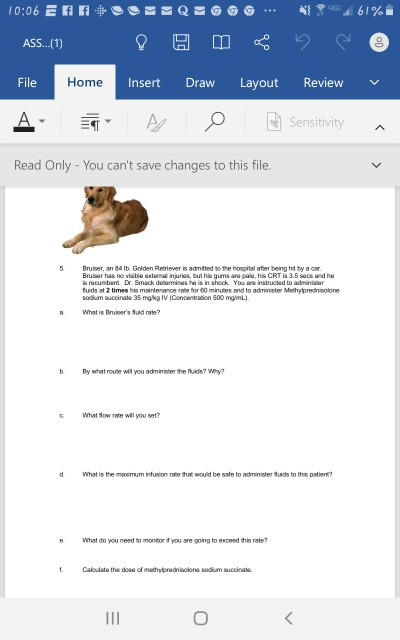I have pages 1-4 that I need help with before 500 pm tommorow. FLUID THERAPY CALCULATIONS FOR Medical Mathamatics Veternarian Nursing Class. 10:06 E FOOTEQ - AS.. (1) 8 g 0 File Home Insert Draw A 7 A 0 N g Layout 661% h e o Review Sensitivity a Read Only - You can't save changes to this file. Bruiser, an 84 lb Golden Retriever is admitted to the hospital after being hit by a car Bruiser has no visible...

• ### i need help with roman numerals asap I is 1 V is 5 X is ten L is 50 C is 100 D is 500 M is 1000 with this you can make any number you can only put 3 of anything in a row though so you can only do III not IIII for 4 you IV if a smaller symbol i

i need help with roman numerals asapI is 1 V is 5 X is ten L is 50 C is 100 D is 500 M is 1000 with this you can make any number you can only put 3 of anything in a row though so you can only do III not IIII for 4 you IV if a smaller symbol is in front then you subtract its worth from the larger. so IL is 49 but if a smaller...

• ### Hi, listed below are questions with explanations on what is needed. really need help with solving...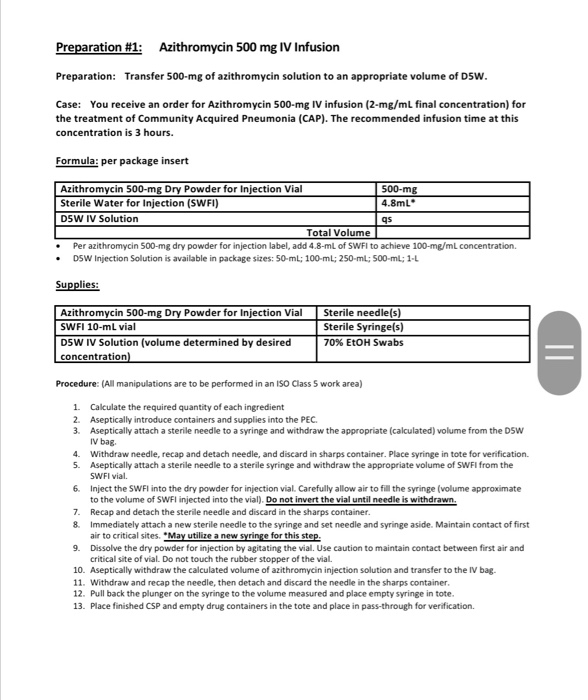Hi, listed below are questions with explanations on what is needed. really need help with solving problems . Preparation #1: Azithromycin 500 mg IV Infusion Preparation: Transfer 500-mg of azithromycin solution to an appropriate volume of D5W. Case: You receive an order for Azithromycin 500-mg IV infusion (2-mg/ml final concentration) for the treatment of Community Acquired Pneumonia (CAP). The recommended infusion time at this concentration is 3 hours. Formula: per package insert Azithromycin 500-mg Dry Powder for Injection Vial 500-mg...

Need Online Homework Help?